# CBSE Solutions for Class 10 Maths

#### Select CBSE Solutions for class 10 Subject & Chapters Wise :

cos 60° cos 30° − sin 60° sin 30° = ?

cos 60o cos 30o − sin 60o sin 30o
=( 1/2 × √3/2 - √3/2 × ½ ) = (√3/4 - √3/4)=0

Evaluate:

sin30°cos45°+cot45°sec60°-sin60°tan45°+cos30°sin90°

sin30°cos45°+cot45°sec60°-sin60°tan45°+cos30°sin90°

=(1/2)(1/√2)+1/2-(√3/2)1+(√3/2)1

=√2/2 + ½ - √3/2 + √3/2

=√2+1/2

sin 60° cos 30° + cos 60° sin 30° = ?

sin 60o cos 30o + cos 60o sin 30o
=(√3/2 × √3/2 + ½ × ½ ) = (3/4 +1/4 )=4/4=1

cos 45° cos 30° + sin 45° sin 30° = ?

cos 45o cos 30o + sin 45o  sin 30o = ?
= (1/√2 × √3/2 + 1/√2 × ½ ) = (√3/2√2 + 1/2√2) = (√3 +1/2√2)

Evaluate:

5cos260°+4sec230°-tan245°sin230°+cos230°

5cos260°+4sec230°-tan245°sin230°+cos230°=

5(1/2)2 + 4(2√3)2 -(1)2(1/2)2+(√3/2)2

=(5/4+4×4/3-1)(1/4+3/4)

=(5/4+16/3-1)(4/4)

=[(15+64-12)/12](4/4)

=(67/12)(1)

=67/12

2 cos2 60° + 3 sin2 45° − 3 sin2 30° + 2 cos2 90° = ?

2 cos2 60o + 3 sin2 45o − 3 sin2 30o + 2 cos2 90o
=2×(1/2)2+3×(1/√2)2-3×(1/2)2 + 2×(0)2

=2×1/4 + 3×1/2- 3×1/4+0

=(1/2+3/2-3/4)
=[(2+6-3)/4]

=54

cot230° − 2cos230° − 3/4sec245° + 1/4 cosec230° = ?

cot2 30o − 2 cos2 30o – 3/4sec2 45o + 1/4cosec230o
= (√3)2-2×(√3/2)2-3/4×(√2)2+1/4×(2)2

= 3-2×3/4-3/4×2+1/4×4

= 3-3/2-3/2+1

= 4-(3/2+3/2)

= 4-3= 1

(sin230° + 4cot245° − sec260°)(cosec245° sec230°) = ?

(sin2 30o + 4 cot2 45o − sec2 60o )(cosec2 45sec2 30o)
=[(1/2)2+4×(1)2-(2)2] [(√2)2 (2/√3)2]=(1/4+4-4) (2×4/3)

=1/4×8/3=2/3

4/cot230°+1/sin230°-2cos245°-sin2 = ?

4cot230° +1sin230° -2 cos245o -sin20o

=4(√3)2+1(1/2)2 - 2×(1/√2)2-(0)2

=4/3+1/4-2×1/2-0

=4/3+4-1 =4/3+3

=(4+9)/3 = 13/3

(1-sin60°)/cos60°= (tan60°-1)/tan60°+1

LHS=1-sin 60ocos 60o=1-√3212=(2-√32)12=(2-√32)×2=2-√3RHS= tan 60o-1tan 60o+1=√3-1√3+1=√3-1√3+1×√3 -1√3 -1=(√3-1)2(√3)2-12=3+1-2√33-1=4-2√32=2-√3

Hence, LHS = RHS

1-sin 60ocos 60o=tan 60o-1tan 60o+1

If sinθ = cos(θ-45°), where θ is acute, then find the value of θ.

We have, sinθ=cos(θ-45°)

cos(90°-θ)=cos(θ-45°)

Comparing both sides, we get

90°- θ = θ - 45°

θ+θ = 90°+45°

2θ=135°

θ=(135/2)°

θ=67.5°

If tanA=5/12, then find the value of (sinA+cosA)secA.                [CBSE 2008]

(sinA+cosA)secA=(sinA+cosA)/cosA=sinA/cosA+cosA/cosA=tanA+1= 5/12+1/1 =(5+12)/12 = 17/12

Write the value of cos1° cos2° ... cos180°.

cos1° cos2° ... cos180°=cos1° cos2° ... cos90° ... cos180°=cos1° cos2° ... 0 ... cos180°=0

Find the value of sin50°/cos40° + cosec40°/sec50°- 4cos50° cosec40°.

sin50°/cos40° + cosec40°/sec50° -4cos50° cosec40° = cos(90°-50°)/cos40° + sec(90°-40°)/sec50° - 4sin(90°-50°) cosec40°

=cos40°/cos40° + sec50°/sec50° - 4sin40°/sin40° = 1+1 – 4 = -2

Find the value of sin48° sec42°+cos48° cosec42°.

sin48° sec42°+cos48° cosec42°= sin48° cosec(90°-42°) + cos48° sec(90°-42°) =sin48° cosec48°+cos48° sec48° =sin48°/sin48° + cos48°/cos48° =1+1 = 2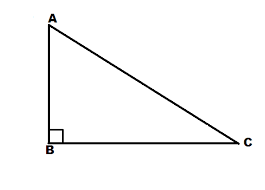1 Sin A A AB/AC 2 Cos A B BC/AC 3 Tan A C BC/AB

1-B, 2-A, 3-C1 Cot A A AC/AB 2 Cosec A B AB/BC 3 Sec A C AC/BC

1-B, 2-C, 3-A

 1 Sin 0° A 32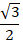2 Sin 30° B 0 3 Sin 45° C ½ 4 Sin 60° D 1/√2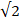1-B, 2-C, 3-D, 4-A

 1 Sin θ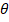A Perpendicular ÷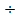Base 2 Cos θB Perpendicular ÷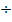Hypotenuse 3 Tan θC Base ÷Hypotenuse

1-B, 2-C, 3-A

 1 Cos 0° A ½ 2 Cos 30° B 1/√23 Cos 45° C 32.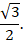4 Cos 60° D 1

1-D, 2-C, 3-B, 4-A